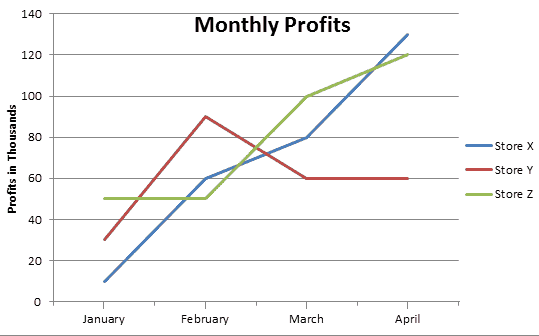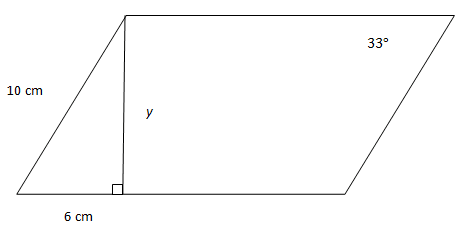# UPCAT Math Practice Test

Last Updated on 10/18/2018 by FilipiKnow

Directions: The Mathematics subtest will test your ability to solve quantitative problems quickly and accurately. Analyze each problem and select the best answer from the multiple choices.

The practice tests are organized around five fundamental math concepts that will be included in the actual UPCAT: arithmetic and number sense, algebra, geometry, trigonometry, and statistics.

To mimic the actual test conditions, you’re not allowed to use calculators. Because UPCAT is a time-pressured exam, we also recommend using a timer when taking the practice test.

1. The average of 7 numbers is 24. The smallest of the numbers is 2 and the largest of the numbers is 31. What is the average of the middle 5 numbers?

A. 25

B. 26

C. 27

D. 28

2. Two side lengths of a triangle are 5 and 6, which of the following CANNOT be the length of the third side?

A. 3

B. 6

C. 9

D. 12

3. Eric wishes to shift the graph of the function ƒ(x) = x² four places to the right and six places down from the origin (0,0). Which equation represents this translation?

A. ƒ(x) = x – 42 + 6

B. ƒ(x) = (x − 4)2 − 6

C. ƒ(x) = (x2 − 4)2 − 6

D. ƒ(x) = x + 42 – 6

4. What is the slope intercept form of (13x − 5x) + 12 − 2y = 6?

A. y = 3x – 9

B. y = 4x + 3

C. y = 6x + 2

D. y = -2x + 5

5. The coordinates (−3, 5) and (3, 5) designate the diameter of a circle, what is its circumference?

A. 2π

B. 4π

C. 6π

D. 8π

6. What is the solution set of  | 2(x – 1) – 15 | = 7?

A. { 5 }

B. { 12 }

C. { 5,12 }

D. { -5,12 }

7. A and B are reciprocals (when multiplied together their product is 1). If A < −1, then B must be which of the following?

A. 0 < B < 1

B. -1 < B < 0

C. B < -1

D. B < 0

8. Which of these is true for all real numbers x and y such that x > 0 and y < 0?

A. xy > 0

B. x + y > 0

C. x2 + y2 > 0

D. x – y < 0

9. At what point on the Cartesian plane do the following two lines intersect?

y/3 – 7/3 = x and y/3 + 4/3 = x

A. (4, 3)

B. (6, 2)

C. The lines do not intersect.

D. (5, -2)

10. The slope of the line represented by the equation 2(x − 3) − 6y = 10 is equal to what?

A. – 1/2

B. 1/3

C. 1/2

D. 2

11. Jenny’s house is 2 km away from her school. One day when going to school, Jenny runs for 20 minutes and arrived 5 minutes late. How many minutes earlier would Jenny be if she would use her bicycle at a rate of one-third kilometer per minute?

A. 5 minutes

B. 6 minutes

C. 8 minutes

D. 9 minutes

12.  The chart below shows the monthly profits of 3 companies. What is the total profit generated by Store X and Store Z in the month of March?A. 120,000

B. 140,000

C. 180,000

D. 200,000

13. Which of the following statements is true?

A. The diagonals of a rhombus are congruent.

B. All rectangles are similar.

C. All rectangles are rhombuses.

D. Some rhombuses are rectangles.

14. In order for the two triangles shown to be similar, what is one possible value for x?A. 8 in.

B. 10 in.

C. 16 in.

D. 20 in.

15. Which coordinates satisfy the inequality: y+ 3 > −3(x − 2)

A. (0,2)

B. (1,0)

C. (-3, 15)

D. (2, -2)

16. If h(x) = 3x + 4, what is h(2x − 3)?

A. -6X + 5

B. 6X – 5

C. 5X – 2

D. 5X + 2

17. If -1 < a < b < 0, then which of the following has the greatest value?

A. b – a

B. a + b

C. a – b

D. 2b – a

18. In the figure below, what is the length of altitude y?A. 7 cm.

B. 8 cm.

C. 9 cm.

D. 10 cm.

19. Which of the following sets of interior angle measures would describe an acute isosceles triangle?

A. 90°, 45°, 45°

B. 80°, 60°, 60°

C. 60°, 60°, 60°

D. 60°, 50°, 50°

20. Which trigonometric function can equal or be greater than 1.000?

A. Sine

B. Cosine

C. Tangent

D. none of the above

FilipiKnow

FilipiKnow strives to ensure each article published on this website is as accurate and reliable as possible. We invite you, our reader, to take part in our mission to provide free, high-quality information for every Juan. If you think this article needs improvement, or if you have suggestions on how we can better achieve our goals, let us know by sending a message to admin at filipiknow dot net

## 15 thoughts on “UPCAT Math Practice Test”

1.Leinad_tawan says:

Thank You! I hope there is a clear explanation and solution to each item?

2. Pingback: UPCAT Math Practice Test | Review Better
3. Pingback: UPCAT MATH PRACTICE TEST | Review Better
4.melissa says:

hello po
how to check if my answers are correct.

thank you

5.Prince ivan says:

Clarification: 15. D. also works.

1.UBT says:

No it wont. Substituting the points from choice D will lead to -1 is greater than (-3)(-4) which is simplified to -1 is greater than positive 12. Now this obviously incorrect

1.Stef says:

How would it be -1 when the coordinates are (2, -2) which means x=2 and y=-2 and substituting that to y+3 > -3(x-2) would make 1>0 which is right, right? Or did I miss some point?

2.Mr.Mozo says:

If inivaluate po natin ung coordinates sa D ito po ang aking sagot
D. (2,-2)
y > -3x+3
-2 > (-3)(2)+3
-2 > -6+3
-2 > -3
True naman ung lumabas na sagot

6.Edward Valdez says:

Need to talk to Mr, Batungbacal by email
Edward Valdez
0956-945-9346
land phone 637-6640

1.Hannah says:

True! I tried answering the letter D as well and it also worked!

7.Shiena says:

Thank you very much! It really helps me

1.FilipiKnow says:

@Shiena

You’re welcome. Good luck on your upcoming exam!

8.Shiena says:

Thank you very much!

9.Andrew Peralta says:

Thank You!

This site uses Akismet to reduce spam. Learn how your comment data is processed.